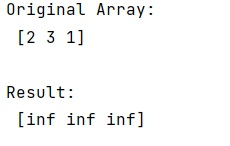# Ignore divide by 0 warning in NumPy

Learn, how to ignore divide by 0 warning in NumPy in Python?
Submitted by Pranit Sharma, on January 19, 2023

NumPy is an abbreviated form of Numerical Python. It is used for different types of scientific operations in python. Numpy is a vast library in python which is used for almost every kind of scientific or mathematical operation. It is itself an array which is a collection of various methods and functions for processing the arrays.

## NumPy - Ignoring divide by 0 warning

Many times there are some calculations that are mathematically not feasible and the compiler generates a warning.

We need to find a way so we can ignore these warnings. For this purpose, we can disable the warning with numpy.seterr(). This method set how floating-point errors are handled. Note that operations on integer scalar types (such as int16) are handled like floating points, and are affected by these settings.

Although it has multiple parameters it has a parameter called divide which if we set it to 'ignore', then will ignore any kind of division warning.

Let us understand with the help of an example,

## Python code to ignore divide by 0 warning in NumPy

```# Import numpy
import numpy as np

# Creating an array
arr = np.array([2, 3, 1])

# Display original array
print("Original Array:\n",arr,"\n")

# Dividing elements of array by 0
np.seterr(divide='ignore')
res = arr/0

# Display result
print("Result:\n",res)
```

Output:What's New (MCQs)

Top Interview Coding Problems/Challenges!

IncludeHelp's Blogs

Languages: » C » C++ » C++ STL » Java » Data Structure » C#.Net » Android » Kotlin » SQL
Web Technologies: » PHP » Python » JavaScript » CSS » Ajax » Node.js » Web programming/HTML
Solved programs: » C » C++ » DS » Java » C#
Aptitude que. & ans.: » C » C++ » Java » DBMS
Interview que. & ans.: » C » Embedded C » Java » SEO » HR
CS Subjects: » CS Basics » O.S. » Networks » DBMS » Embedded Systems » Cloud Computing
» Machine learning » CS Organizations » Linux » DOS
More: » Articles » Puzzles » News/Updates